# Charting#

## chart keywords#

ahlive has these keywords for various types of charts:

ahlive keyword

description

line

connect data coordinates with a line

scatter

mark data coordinates with symbols

bar

fill data coordinates with vertical rectangles

barh

fill data coordinates with horizontal rectangles

pie

fill data arrays with fractional wedges

errorbar

connect data coordinates with a line plus segments

area

fill data coordinates with regions

annotation

label data coordinates with text

pcolormesh

paints data grids with filled colors

contourf

contour data grids with filled colors

contour

contour data grids with line colors

hexbin

groups data grids as 2D histograms

quiver

draws data grids as arrows

streamplot

draws data grids as streamlines

windbarb

draws data grid as wind barbs

A chart’s behavior may be modified by setting a preset. Learn more in Presetting.

## line#

Draws a line, segment by segment, connecting the data points.

:

import ahlive as ah
ah.Array([-5, 0, 5], [1, -1, 0.5], xlims="fixed", chart="line").render()

: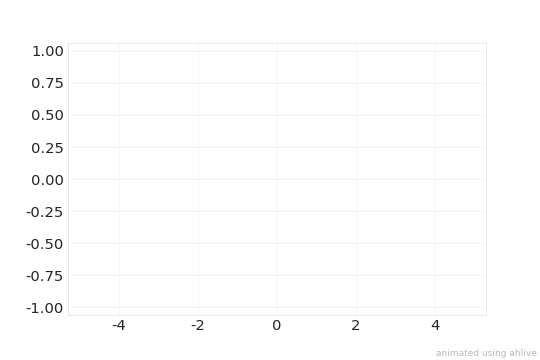## scatter#

Draws a symbol that moves one coordinate point at a time.

:

import ahlive as ah
ah.Array([-5, 0, 5], [1, -1, 0.5], xlims="fixed", chart="scatter").render()

gifsicle: warning: trivial adaptive palette (only 210 colors in source)

: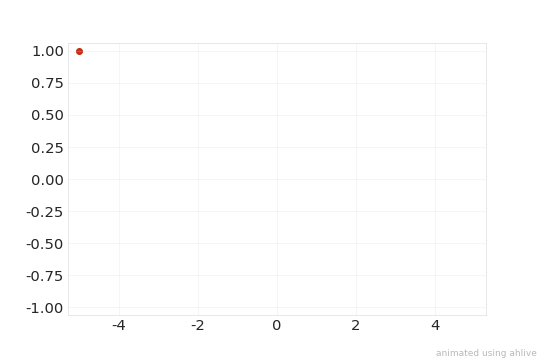## bar#

Draws bars, fusing the data points.

:

import ahlive as ah
ah.Array([-5, 0, 5], [1, -1, 0.5], xlims="fixed", chart="bar").render()

gifsicle: warning: trivial adaptive palette (only 199 colors in source)

: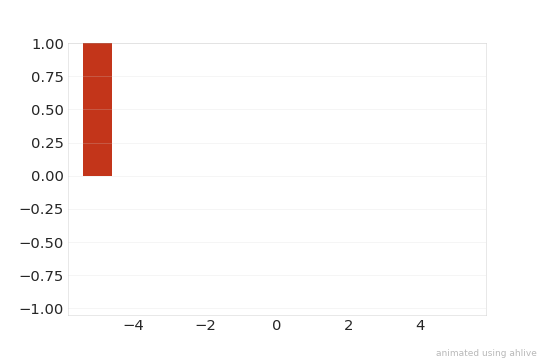## barh#

Same as bar, but horizontally.

:

import ahlive as ah
ah.Array([-5, 0, 5], [1, -1, 0.5], xlims="fixed", chart="barh").render()

gifsicle: warning: trivial adaptive palette (only 182 colors in source)

: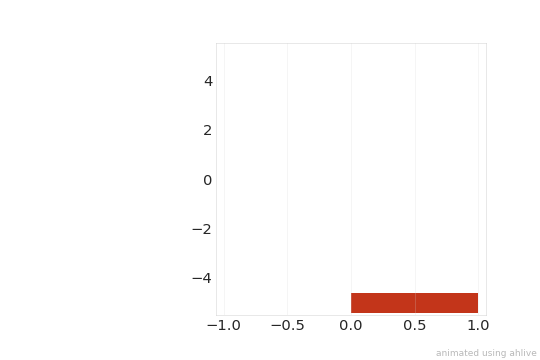## pie#

Draws a fractionated circle with the area representing the data points.

:

import ahlive as ah
ah.Array([0.5, 0.75, 1], chart="pie").render()

: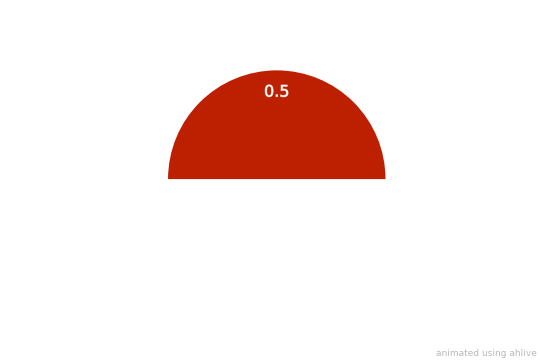If both xs and ys are passed, xs will be ignored.

## errorbar#

Like line, but with vertical or horizontal segments at each base frame.

:

import ahlive as ah
ah.Array(
[0, 1, 2],
[1, 2, 3],
yerrs=[0.25, 0.5, 0.1],
xlims="fixed",
chart="errorbar",
).render()

: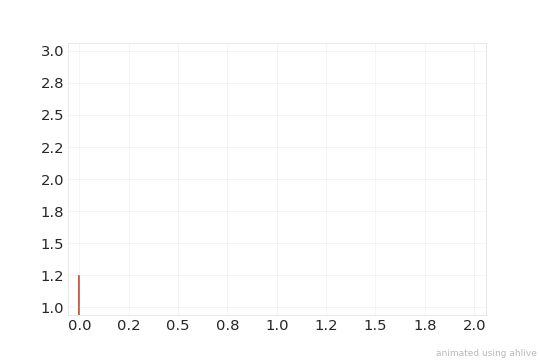## area#

Fills in regions, step by step.

:

import ahlive as ah
ah.Array(
[0, 1, 2],
[3, 2, 1.5],
y2=[2, 1.5, 1.5],
xlims="fixed",
chart="area",
).render()

: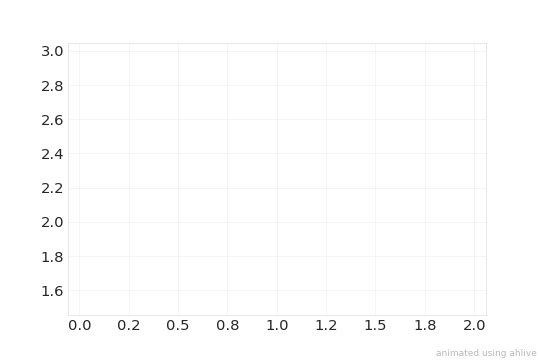## annotation#

Have a label follow data coordinates.

:

import ahlive as ah
ah.Array(
[0, 1, 2],
[1, 2, 1.5],
text=["a", "b", "c"],
xlims="fixed",
chart="annotation"
).render()

gifsicle: warning: trivial adaptive palette (only 205 colors in source)

: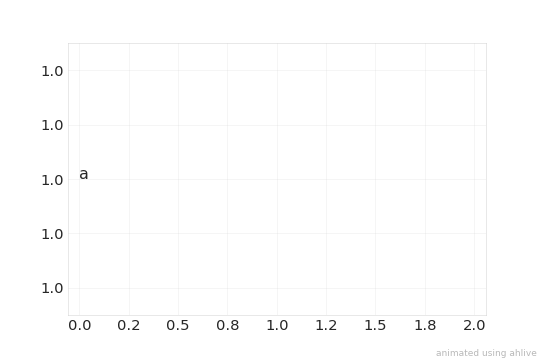## pcolormesh#

Maps color to each grid cell based on the value.

:

import ahlive as ah
import numpy as np

xs, ys = np.arange(0, 2 * np.pi, .5), np.arange(0, 2 * np.pi, .5)
X, Y = np.meshgrid(xs, ys)
cs = np.stack([np.sin(X), np.cos(X)]) * np.stack([np.sin(Y), np.sin(Y)])

ah.Array2D(xs, ys, cs=cs, chart="pcolormesh").render()

gifsicle: warning: trivial adaptive palette (only 203 colors in source)

: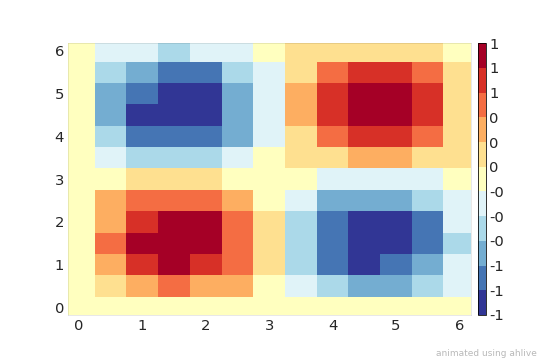## contourf#

Computes discrete contours and maps color to each interval.

:

import ahlive as ah
import numpy as np

xs, ys = np.arange(0, 2 * np.pi, .5), np.arange(0, 2 * np.pi, .5)
X, Y = np.meshgrid(xs, ys)
cs = np.stack([np.sin(X), np.cos(X)]) * np.stack([np.sin(Y), np.sin(Y)])

ah.Array2D(xs, ys, cs=cs, chart="contourf").render()

/home/docs/checkouts/readthedocs.org/user_builds/ahlive/conda/main/lib/python3.9/site-packages/ahlive-1.0.0.post14+dirty-py3.9.egg/ahlive/data.py:916: UserWarning: num_colors is ignored for contourf!

gifsicle: warning: trivial adaptive palette (only 211 colors in source)

: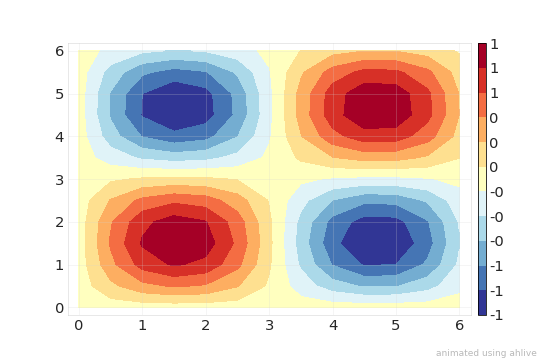## contour#

Like contourf, but not filled.

:

import ahlive as ah
import numpy as np

xs, ys = np.arange(0, 2 * np.pi, .5), np.arange(0, 2 * np.pi, .5)
X, Y = np.meshgrid(xs, ys)
cs = np.stack([np.sin(X), np.cos(X)]) * np.stack([np.sin(Y), np.sin(Y)])

ah.Array2D(xs, ys, cs=cs, chart="contour").render()

: